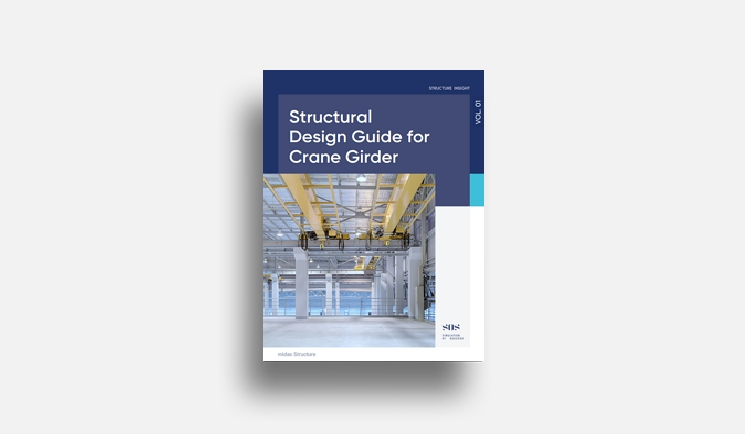# midas Structure

BLOG NEWS & TRENDS

OVERVIEW

The P-Delta analysis is a type of Geometric nonlinearity, which accounts for secondary structural behavior when axial and transverse loads are simultaneously applied to beam or wall elements. The P-Delta effect is more profound in tall building structures where high vertical axial forces act upon the laterally displaced structures caused by high lateral forces.

Virtually all design codes such as ACI 318 and AISC-LRFD specify that the P-Delta effect be included in structural analyses to account for more realistic member forces.

The P-Delta analysis feature in midas Gen & nGen is founded on the numerical analysis method adopted for Buckling analysis. Linear static analysis is performed first for a given loading condition. Then a new geometric stiffness matrix is formulated based on the member forces or stresses obtained from the first analysis. Thus, the geometric stiffness matrix is repeatedly modified and used to perform subsequent static analyses until the given convergence conditions are satisfied.

As shown in Figure 1, static loading conditions are also required to consider the P-Delta effect for dynamic analyses.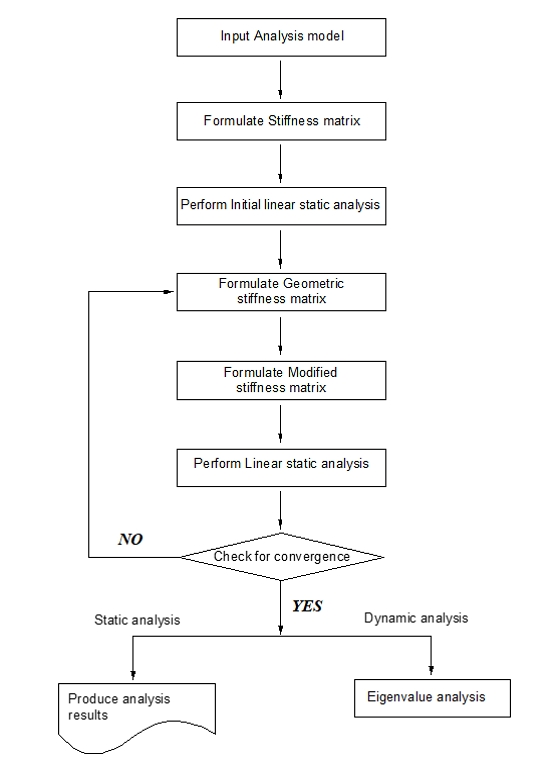Figure 1 Flow chart for P-Delta analysis in MIDAS/Gen

When a lateral load acts upon a column member, thereby resulting in moments and shear forces in the member, an additional tension force reduces the member forces. In contrast, another compression force increases the member forces.

Accordingly, tension forces acting on column members subjected to lateral loads increase the stiffness pertaining to lateral behaviors, while compression forces have an opposite effect on the column members.

Objective

Compare the Analysis results with/without P-Delta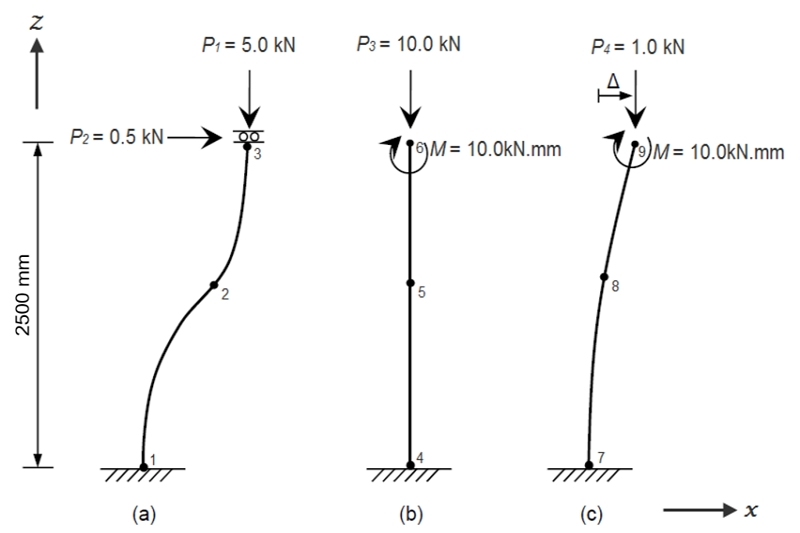1. Define Material and Section

Define Material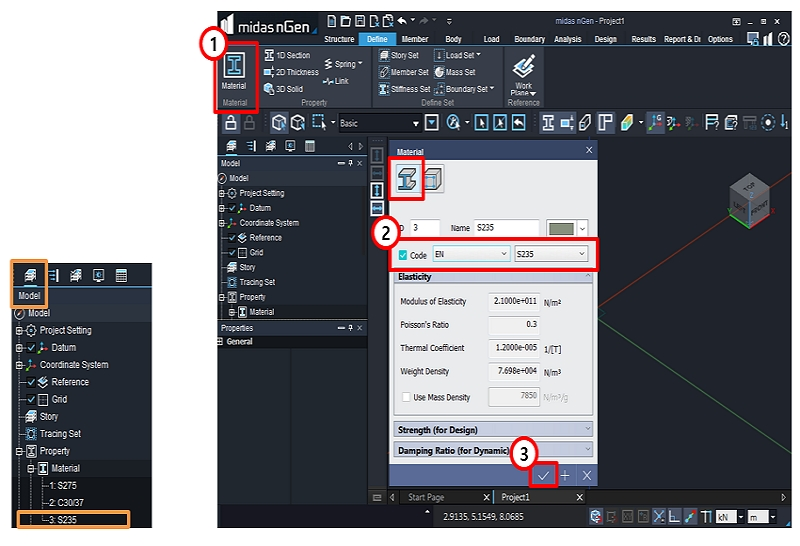1. select [Define>Material].

2. Select [EN] and [S235].

3. Click [OK].

Confirm from Model Tree Menu [Property>Material]

Define 1D Selection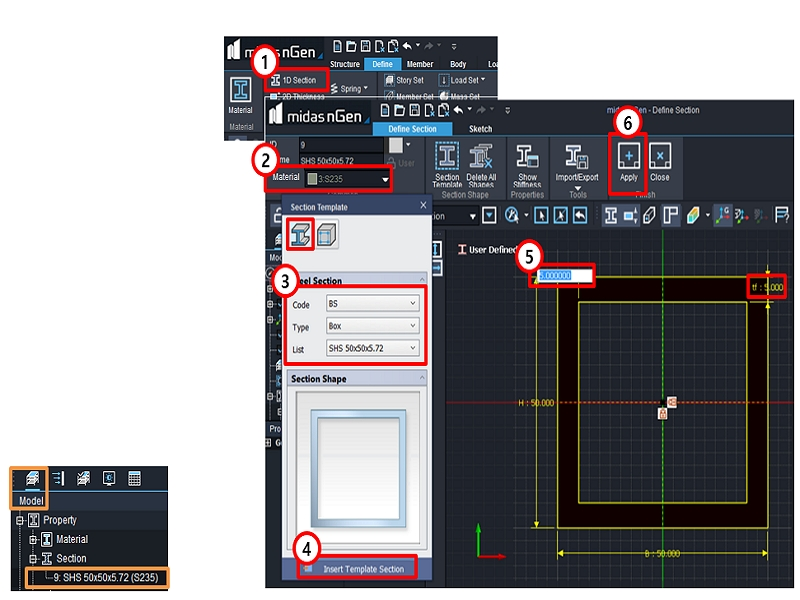1. select [ Define > 1D Selection ].

2. Select [ Material > S235].

3. Select Code : [ BS ].

Type : [ Box ].

List : [SHS 50*50*5.72 ].

4. Click [ Insert Template Section ].

5. Enter [ 5 ] to modify the width tw and tf > Press [ Enter ].

6. Click [ Apply ] & [ Close ].

Confirm from Model Tree Menu [ Property > Section ].

2. Create Columns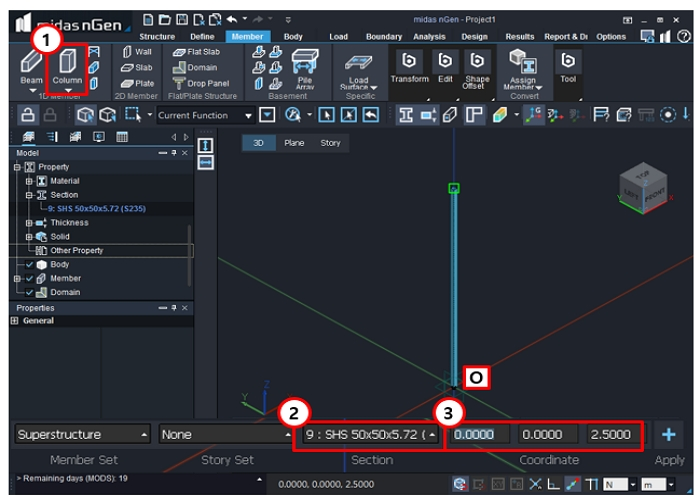1. Select [ Member > Column ].

2. Select [ SHS 50*50*5.72 (235) ].

3. Click at Datum Origin [ 0 ] > Enter [ 0, 0, 2.5] > Press [ Enter ] > Press [ Esc ] to finish.

3. Copy Columns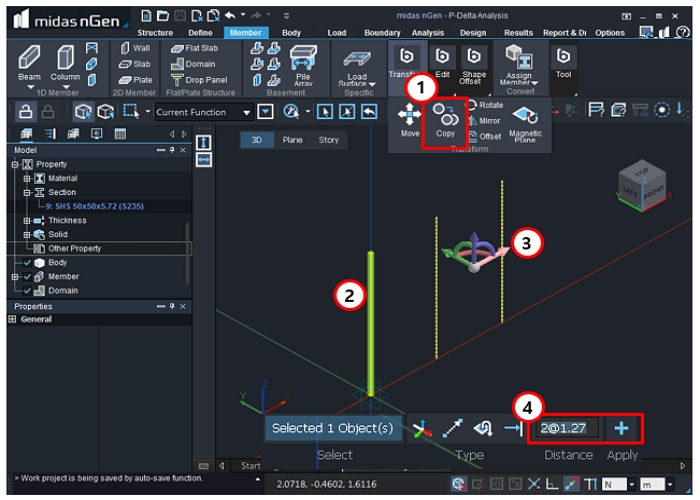1. Select [ Member > Copy ].

2. Select the Column.

3. Select [X-axis ].

4. Enter [2@1.27] > Click [ Apply ].

5. Press [ Esc ] to finish.

4. Define Supports

Define Support - 1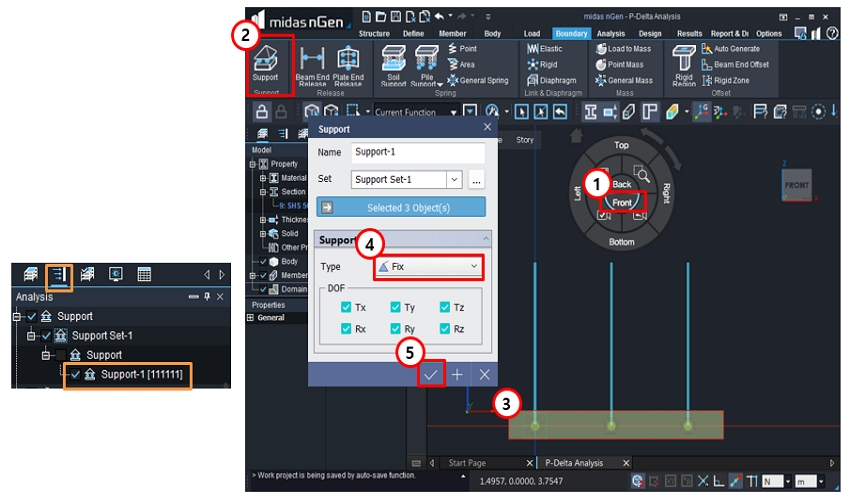Apply supports

1. Go to [ Front ] view.

2. Select [ Boundary > Support ].

3. Select [ the ends of 3 columns ].

4. Select [ Fix ] > Select [ All DOF ].

5. Click [ OK ].

Confirm [ Work Tree > Analysis > Support > Support - 1 ].

Define Support - 2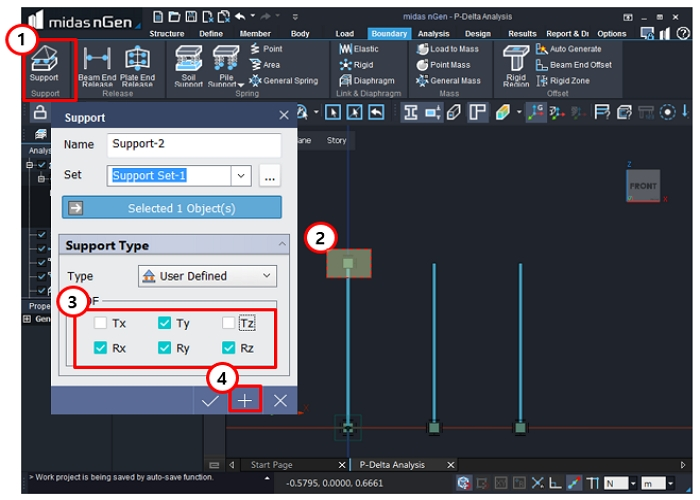Apply supports

1. Select [ Boundary > Support ].

2. Select the [ top of 1st column ].

3. Check off [ Tx] & [Tz ].

4. Click [ Apply ].

Define Support - 3 & 4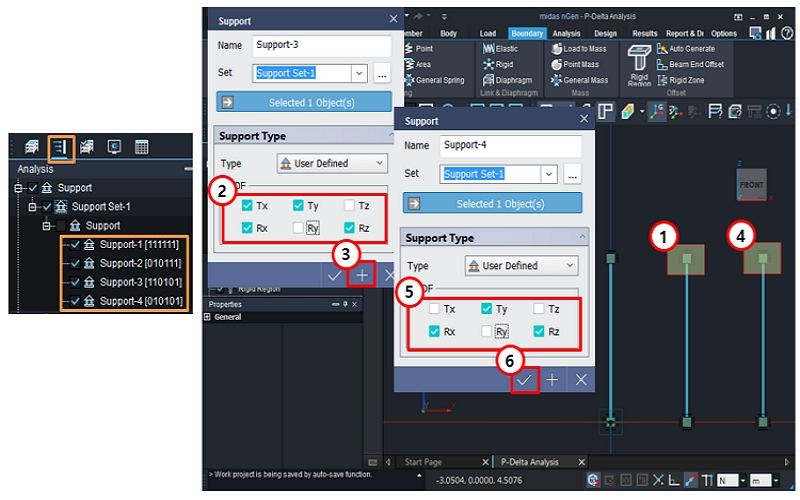Apply supports

1. Select the [ top of 2nd column ].

2. Check on [ Tx ] & Check off [ Ry ].

3. Click [ Aplply ].

4. Select the [ top of 3rd column ].

5. Check off [ Tx ], [ Tz ], [ Ry ].

6. Click [ OK ].

Confirm [ Work Tree > Analysis > Support > Support Set - 1 ].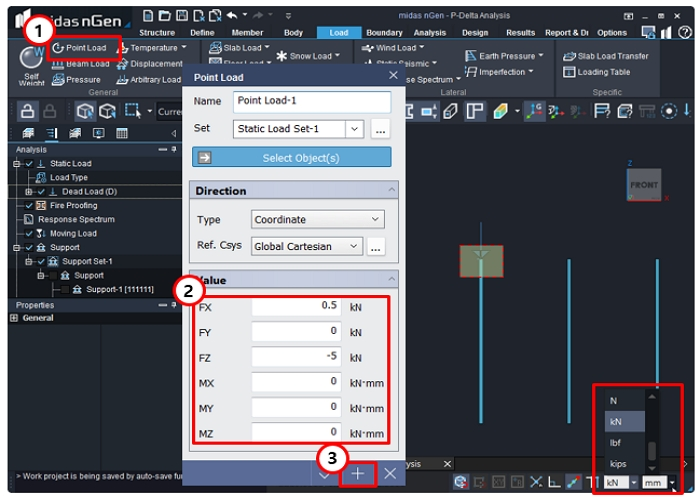Apply supports

2. Select point > Enter [ 0.5 ]  in  'FX' > Enter [ -5 ] in 'FZ'.

3. Click [ Apply ].

Apply Point Load - 2 & 3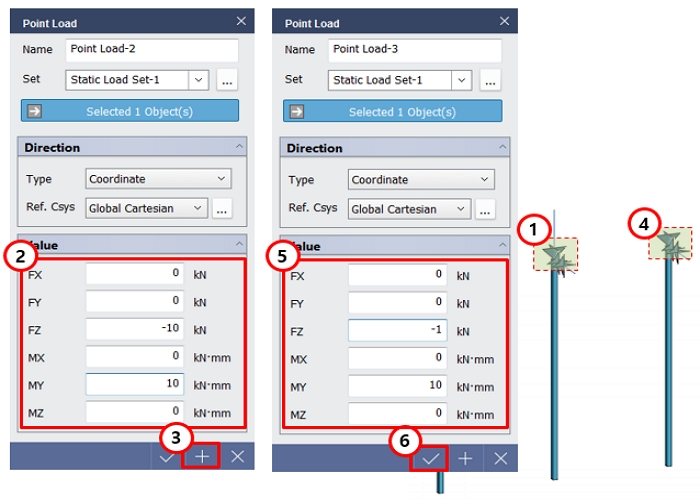Apply Point Load - 2 & 3

1. Select point.

2. Enter [ 0 ] in 'FX' > Enter [ -10 ] in 'FZ' > Enter [ 10 ] in 'MY'.

3. Click [ Apply ].

4. Select point.

5. Enter [ 0 ] in 'FX' > Enter [ -10 ] in 'FZ' > Enter [ 10 ] in 'MY'.

6. Click [ OK ].

6. Perform Analysis

Analysis Settings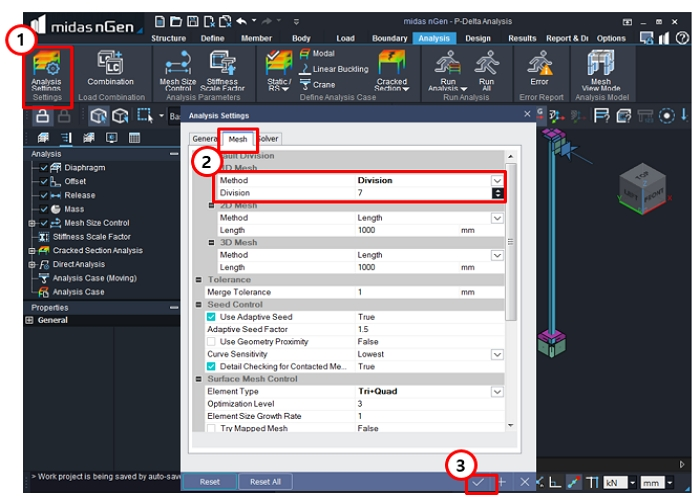Analysis Settings

1. Select [ Analysis > Analysis Settings ].

2. Select [ Mesh ] > Enter '7' in Division.

3. Click [ OK ].

Perform Analysis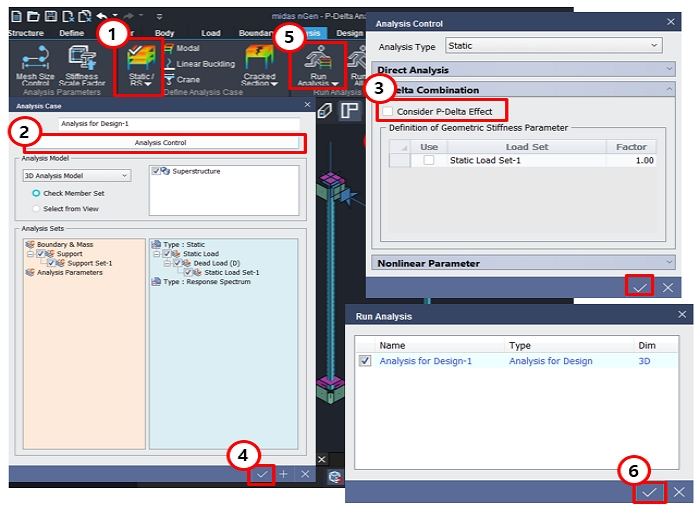Define Analysis Case & Run Analysis

1. Select [ Analysis > Static/RS ].

2. Click [ Analysis Control ].

3. Check off [ P-Delta ] > Click [ OK ].

4. Click [ OK ].

5. Select [ Run Analysis ].

6. Click [ OK ].

7. Analysis Results

Confirm Analysis Results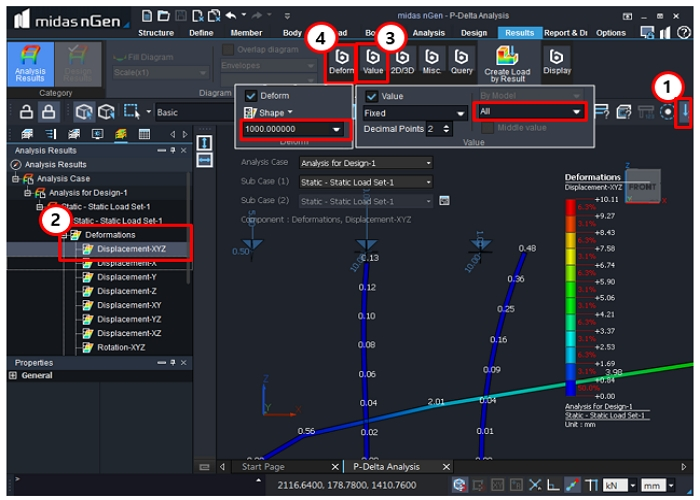Deformation

1. Click Indicator 1. [ Show/Hide Load Value ].

2. Select [ Deformation > Displacement-XYZ ].

3. Check on [ Value ] > Select [ Fixed > All ].

4. Check on [ Deform ] > Define Scale [ x1, x0.5, x2 ] > User [ 1000 ].

Subtle deformation occurs at 2nd column since [ Ry ] was deselected at top support.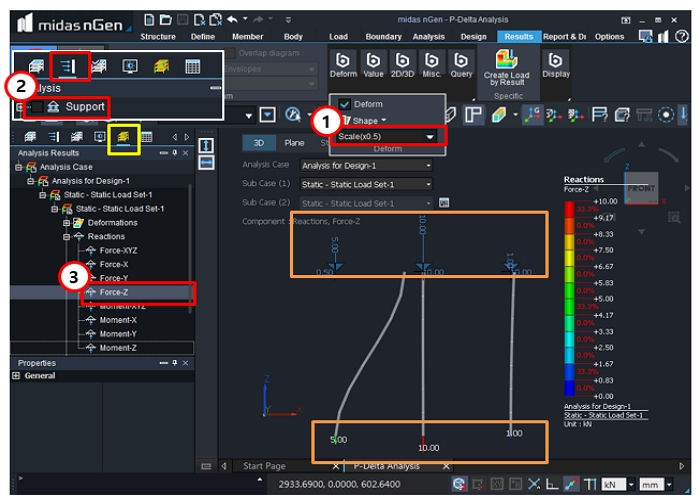Reaction

1. Adjust the scale of [ Deform ].

2. Click Indicator 2. > Check off [ Support ].

3. Click the Yellow box > Select [ Reactions > Force-Z ].

Reaction Force-Z and Load-Z are the same.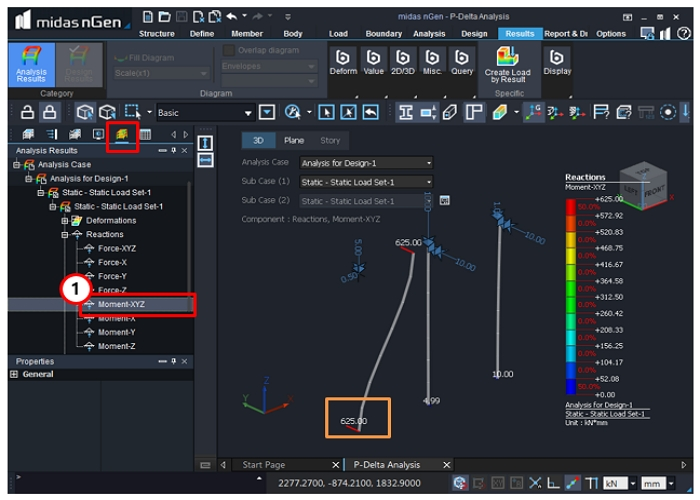Reaction

1. Select [ Reactions > Moment-XYZ ].

645 kN.MM = 0.5kN * 2500mm/2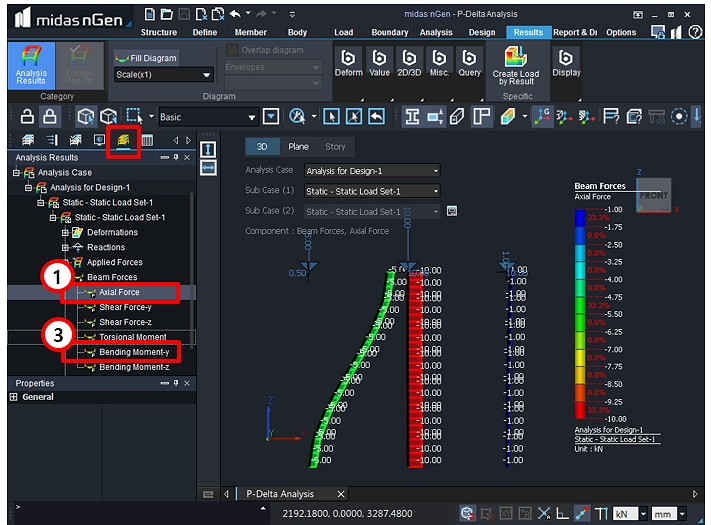Beam Forces

1. Select [ Beam > Force > Axial Force ].

2. Axial Force and Load-Z are the same.

3. Select [ Beam > Force > Bending Moment-y ].

Check Bending moment-y induced by deformation.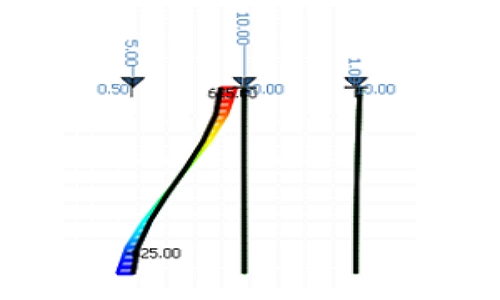8. Perform P-Delta Analysis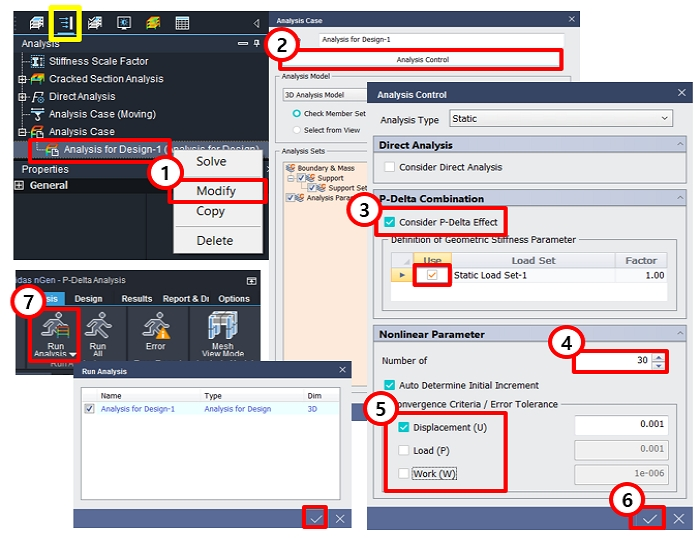P-Delta Analysis Case

1. Click the Yellow Box > Select [ Analysis for Design-1 ] > Right-click >

Click [ Modify ].

2. Click [ Analysis Control ].

3. Select [ Consider P-Delta Effect > Static Load Set-1 ].

4. Enter [ 30 ] in Number of Increments.

5. Select [ Displacement ] > Check off [ Load > Work ].

6. Click [ OK ] > [OK ] > [ YES ].

7. Select [ Analysis > Run Analysis ].

9. P-Delta Analysis Result

Confirm Analysis Results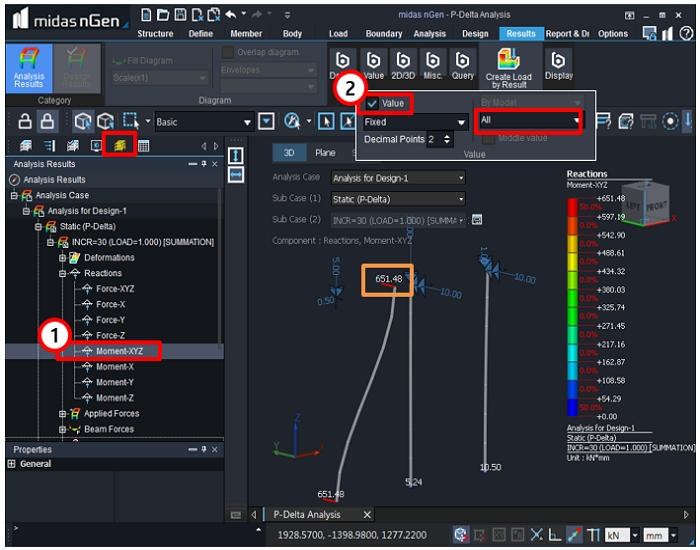Reactions

1. Select [ Reactions > Moment-XYZ ].

2. Select [ Value > Fixed > All ].

Reaction moments have increased due to P-Delta Effect.